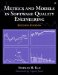# Model Assumptions

Reliability modeling is an attempt to summarize the complex reality in precise statistical terms. Because the physical process being modeled (the software failure phenomenon ) can hardly be expected to be so precise, unambiguous statements of the assumptions are necessary in the development of a model. In applications, the models perform better when the underlying assumptions are met, and vice versa. In other words, the more reasonable the assumptions, the better a model will be. From the preceding summary of several reliability growth models, we can see that earlier models tend to have more restrictive assumptions. More recent models tend to be able to deal with more realistic assumptions. For instance, the J-M model's five assumptions are:

1. There are N unknown software faults at the start of testing.
2. Failures occur randomly ”times between failures are independent.
3. All faults contribute equally to cause a failure.
4. Fix time is negligible.
5. Fix is perfect for each failure; there are no new faults introduced during correction.

Together these assumptions are difficult to meet in practical development environments. Although assumption 1 does not seem to pose problems, all the others pose limitations to the model. The Littlewood models, with the concept of error size , overcame the restriction imposed by assumption 3. The Goel-Okumoto imperfect debugging model is an attempt to improve assumptions 4 and 5.

Assumption 2 is used in all time between failures models. It requires that successive failure times be independent of each other. This assumption could be met if successive test cases were chosen randomly. However, the test process is not likely to be random; testing, especially functional testing, is not based on independent test cases. If a critical fault is discovered in a code segment, the tester may intensify the testing of associated code paths and look for other faults. Such activities may mean a shorter time to next failure. Strict adherence to this assumption therefore is not likely. Care should be taken, however, to ensure some degree of independence in data points when using the time between failures models.

The previous assumptions pertain to the time between failures models. In general, assumptions of the time between failures models tend to be more restrictive. Furthermore, time between failures data are more costly to gather and require a higher degree of precision.

The basic assumptions of the fault count model are as follows (Goel, 1985):

1. Testing intervals are independent of each other.
2. Testing during intervals is reasonably homogeneous.
3. Numbers of defects detected during nonoverlapping intervals are independent of each other.

As discussed earlier, the assumption of a homogeneous testing effort is the key to the fault count models. If this assumption is not met, some normalization effort or statistical adjustment should be applied. The other two assumptions are quite reasonable, especially if the model is calendar-time based with wide enough intervals (e.g., weeks).

For both classes of models, the most important underlying assumption is that of effective testing. If the test process is not well planned and test cases are poorly designed, the input data and the model projections will be overly optimistic. If the models are used for comparisons across products, then additional indicators of the effectiveness or coverage of testing should be included for the interpretation of results.Metrics and Models in Software Quality Engineering (2nd Edition)
ISBN: 0201729156
EAN: 2147483647
Year: 2001
Pages: 176